﻿ 多孔材料孔径分布测试方法的研究 Analysis Method of Pore Size Distribution of Porous Materials

Material Sciences
Vol. 10  No. 02 ( 2020 ), Article ID: 34180 , 9 pages
10.12677/MS.2020.102012

Analysis Method of Pore Size Distribution of Porous Materials

Lixia Bao, Peifeng Gao, Shaochun Peng

Analysis and Testing Center, Beijing Institute of Technology, Beijing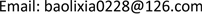Received: Jan. 22nd, 2020; accepted: Feb. 6th, 2020; published: Feb. 13th, 2020ABSTRACT

Pore size and pore size distribution are one of the important properties of porous materials. Various theoretical models for pore size analysis, including Barrett-Joyner-Halenda (BJH), Horvath-Kawazoe (HK), Saito-Foley (SF) and NLDFT (non-local density functional theory) are introduced, and the applicable conditions of each theoretical model are summarized. The results show that BJH method can be used to analyze cylindrical mesoporous materials with pore size between 5 nm and 50 nm. HK method is more suitable for the analysis of microporous activated carbon materials with slit holes, and SF method is intended for analyzing cylindrical microporous materials according to the adsorption isotherms determined by argon. NLDFT method is more suitable for the analysis of micro-mesoporous composites, which has a wide range of applications and prospects.

Keywords:Pore Size Distribution, Porous Materials, BJH, HK, SF, NLDFT1. 引言

2. 介孔材料孔径分析方法

Barrett-Joyner-Halenda (BJH)方法基于Kelvein和Halsey方程，是最早确定孔径分布的方法之一。Kelvin方程以热动力学为基础，方程形式如下 

$\mathrm{ln}\frac{P}{{P}_{0}}=-\frac{2\gamma \stackrel{¯}{V}}{\gamma RT}\mathrm{cos}\theta$ (1)

P是液体在半径为r的微孔中的平衡蒸汽压，P0是液体的饱和蒸汽压力，γ和 $\stackrel{¯}{V}$ 分别是液体的表面张力和摩尔体积，θ是液体在微孔中的接触角。r是Kelvin半径或者临界半径，不是微孔的真正半径，假设发生凝聚时吸附层厚度为t，则微孔的真正孔半径可表示为

${\gamma }_{P}=\gamma +t$ (2)

t由Halsey方程确定，

$t=\tau {\left(\frac{5}{2.303\mathrm{log}\frac{{P}_{0}}{P}}\right)}^{\frac{1}{3}}$ (3)

$\tau$ 是单吸附层厚度。当吸附质为氮气时， $\tau =0.354\text{\hspace{0.17em}}\text{nm}$，带入(3)即可得到吸附层厚度与压力的平衡关系式：

$t=0.354{\left[-\frac{5}{\mathrm{ln}\left(P/{P}_{0}\right)}\right]}^{\frac{1}{3}}$ (4)

BJH法是应用Kelvin方程通过简单的几何计算分析孔径的经典方法，它假设孔型是圆柱孔，仅适用于指定的孔径范围并且低估了孔径，容易导致重大偏差(孔径 < 5 nm时，偏差可达25%)，主要适用于大于5 nm的介孔柱状模型 。ISO15901《固体材料孔径分布与孔隙率的压汞法和气体吸附法测定–第2部分：气体吸附法分析介孔和宏孔》对BJH的使用提出了明确的限定条件：1) 孔隙是刚性的，并且有规则的形状(比如，圆柱状)；2) 不存在微孔；3) 孔径分布不连续超出此方法所能测定的最大孔隙，即在最高相对压力处，所有测定的孔隙均已被充满。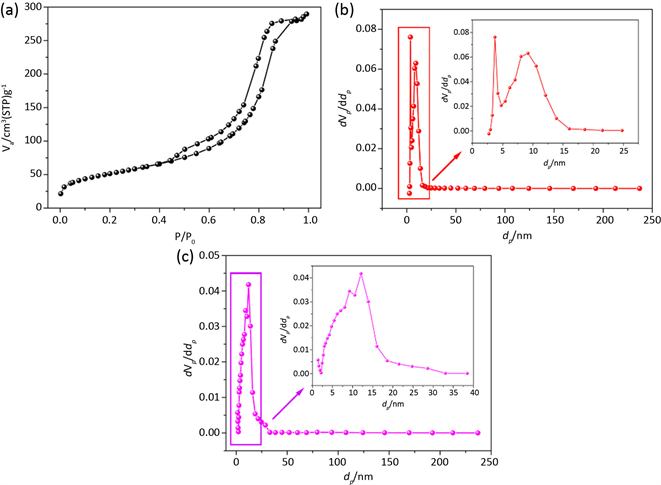Figure 1. BJH Pore Size Distribution (PSD) of Alumina (a-Adsorption Isotherm; b-PSD by Adsorption Branch; c-PSD by desorption Branch)

3. 微孔材料孔径分析方法

BJH方法可应用于孔径大于5 nm的介孔材料孔径分析，但不适用于微孔填充的描述  。主要原因在于，其一，Kelvin方程在孔径小于2 nm时是不适用的；其二，毛细凝聚现象描述的孔中的吸附质为液态，而在微孔中，由于密集孔壁的交互作用，使得填充于微孔中的吸附质处于非液态状态。因此，BJH法不再适用于微孔孔径分析。

3.1. Horvath-Kawazoe (HK)法

HK法  是一种由微孔上样品氮吸附等温线计算有效孔径分布的半经验分析方法。该方法假设材料为狭缝孔(这些孔多出现在炭分子筛和活性炭内)。Horvath和Kawazoe通过热力学讨论，发现平均势能与吸附的自由能变有关，得出了微孔内吸附能与有效孔径之间的关系：

$RT\mathrm{ln}\left(\frac{P}{{P}_{0}}\right)=K\frac{{N}_{S}{A}_{S}+{N}_{A}{A}_{A}}{{\sigma }^{4}\left(l-d\right)}\left[\frac{{\sigma }^{4}}{3{\left(l-\frac{d}{2}\right)}^{3}}-\frac{{\sigma }^{10}}{9{\left(l-\frac{d}{2}\right)}^{9}}-\frac{{\sigma }^{4}}{3{\left(\frac{d}{2}\right)}^{3}}+\frac{{\sigma }^{10}}{9{\left(\frac{d}{2}\right)}^{9}}\right]$ (5)

${A}_{S}=\frac{6m{c}^{2}{a}_{S}{a}_{A}}{\frac{{a}_{S}}{{x}_{S}}+\frac{{a}_{A}}{{x}_{A}}}$ (6)

${N}_{A}$ 为吸附质每单位面积上的分子数，m是电子的质量，c为光速， ${a}_{S}$ 是吸附剂的极化率， ${a}_{A}$ 是吸附质的极化率， ${x}_{S}$ 是吸附剂的磁化率， ${x}_{A}$ 是吸附质的磁化率。

${A}_{A}=\frac{3m{c}^{2}{a}_{S}{x}_{A}}{2}$ (7)

$d={d}_{S}+{d}_{A}$ (8)

${d}_{S}$ 是吸附剂分子的直径， ${d}_{A}$ 是吸附质分子的直径，l是吸附剂上两层吸附质之间的距离， $\sigma =\frac{0.858d}{2}$ 由式(5)可以看出对于某一给定尺寸和形状的微孔会在某一特定相对压力下发生微孔填充，该特征压力直接与吸附剂–吸附质相互作用有关。

HK法适用于狭缝孔微孔炭材料，根据液氮温度下的氮气吸附等温线进行孔径分析，分析结果也更接近于实际数值。将该法应用于沸石分子筛微孔孔径分析中，是错误的。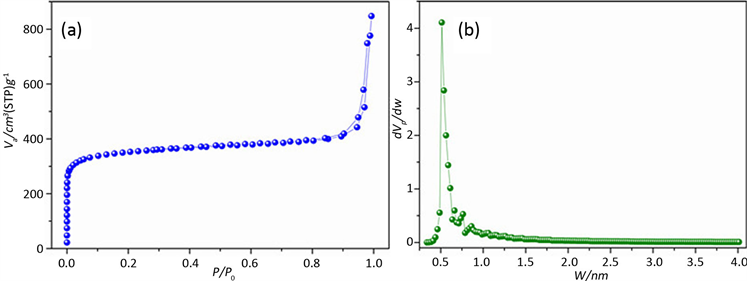Figure 2. PSD of Carbon Matirial by HK (a-Adsorption Isotherm; b-PSD by HK)

3.2. Saito-Foley (SF)法

Saito和Foley将HK法扩展到由氩87 K时在沸石分子筛上的吸附等温线计算有效孔径分布。Saito和Foley (SF)法同样基于Everett和Powl势能方程，假设孔型为圆柱形。HK对数运算式，Saito和Foley导出一类似于HK方程的关系式，其将微孔填充的相对压力与(有效)孔径 ${d}_{p}=l-{d}_{s}$ 相关联。

$\mathrm{ln}\left(\frac{p}{{p}_{0}}\right)=\frac{3}{4}K\frac{\pi {N}_{A}}{RT}\frac{{N}_{S}{A}_{S}+{N}_{A}{A}_{A}}{{d}_{0}^{4}}{f}_{SF}\left(\alpha ,\beta ,l,{d}_{0}\right)$ (9)

${f}_{SF}\left(\alpha ,\beta ,l,{d}_{0}\right)=\underset{k=0}{\overset{\infty }{\sum }}\left[\frac{1}{1+k}{\left(1-\frac{2{d}_{0}}{l}\right)}^{2k}\left\{\frac{21}{32}{\alpha }_{k}{\left(\frac{2{d}_{0}}{l}\right)}^{10}-{\beta }_{k}{\left(\frac{2{d}_{0}}{l}\right)}^{4}\right\}\right]$ (10)

${\alpha }_{k}={\left(\frac{-4.5-k}{k}\right)}^{2}{\alpha }_{k-1}$ (11)

${\beta }_{k}={\left(\frac{-1.5-k}{k}\right)}^{2}{\beta }_{k-1}$ (12)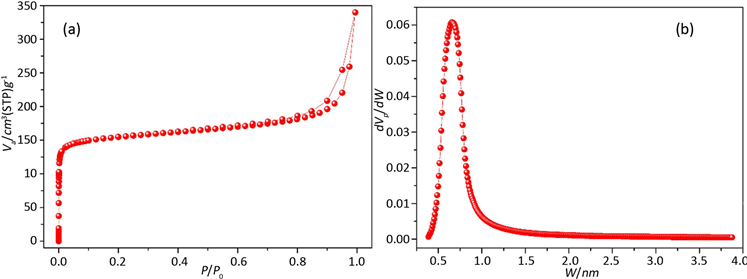Figure 3. PSD of Zeolite by SF (a-Adsorption Isotherm; b-PSD by SF)

4. 微介孔材料孔径分析方法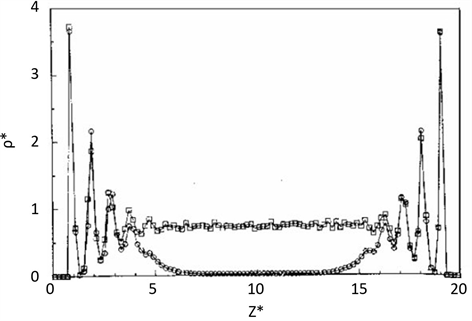Figure 4. Density distribution of coexisting gas (spherical) and liquid (square) in 20 molecular diameter fracture pores 

NLDFT法假设吸附等温线是由无数个别的“单孔”吸附等温线乘以它们的覆盖孔径范围的相对分布得到的。只要给定吸附质/吸附剂的体系，就能通过DFT或MC模拟得到一组等温线(也叫Kernel，即核心文件或影响函数)，通过快速负数最小二乘法解方程就能推导出孔径分布曲线。NLDFT可以比较实验等温线和计算等温线，对等温线的拟合非常好，则表明反映孔径状态准确。用于碳、二氧化硅、沸石等各种材料类型的不同孔模型已经开发并且得到广泛应用(例如，狭缝孔、筒型孔和球形孔以及混合孔形)。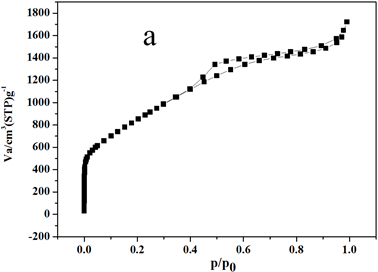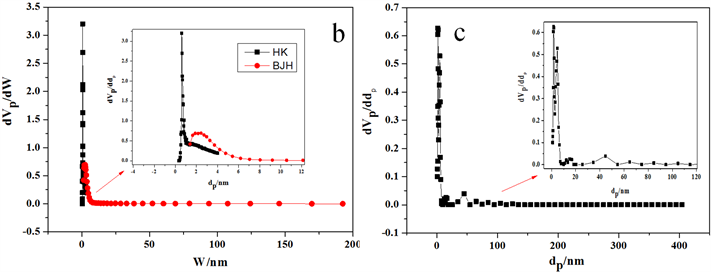Figure 5. PSD of micro-mesoporous carbon materials (a-Adsorption Isotherm; b-BJH-HK method; c-NLDFT method)

5. 总结

1) 多孔材料在进行孔径分析时，要选择合适的理论模型，才能够得出准确的结果。

2) 介孔材料，如果是圆柱型孔或者接近圆柱型孔的，可以选择BJH理论进行孔径分析，若是孔径大于5 nm的介孔材料，孔径分析的结果更准确。

3) HK理论模型适用于狭缝孔的微孔炭材料孔径分析，分析结果比较准确；SF法更适用于圆柱型的微孔分子筛材料，需要基于微孔材料氩气吸附的等温线进行孔径分析。

4) 对于微介孔材料，选择NLDFT理论分析结果是最准确的。NLDFT理论可以用于全孔分析，在选择Kenel文件时，要尽量保证孔型与材料相符，保证模拟吸附等温线与实验吸附等温线误差最小，才能提高分析结果的准确性。

Analysis Method of Pore Size Distribution of Porous Materials[J]. 材料科学, 2020, 10(02): 95-103. https://doi.org/10.12677/MS.2020.102012

1. 1. Ying, J.Y., Mehner, C.P. and Wong, M.S. (1999) Synthesis and Applications of Supramolecular-Templated Mesoporous Materials. Angewandte Chemie International Edition, 38, 56 -77.

2. 2. 闫继娜, 施剑林, 陈航榕, 等. 纳米介孔材料的催化应用前景[J]. 无机材料学报, 2003, 18(4): 725-730.

3. 3. 侯梅芳, 崔杏雨, 李瑞丰. 沸石分子筛在气体吸附分离方面的应用研究[J]. 太原理工大学学报, 2001, 32(2): 135-139 .

4. 4. Yu, J.C., Wang, X. and Fu, X. (2004) Pore-Wall Chemistry and Photocatalytic Activity of Mesoporous Titania Molecular Sieve Films. Chemistry of Materials, 16, 1523-1530. https://doi.org/10.1021/cm049955x

5. 5. 刘欣梅, 阎子峰, Lu, G.Q. 介孔纳米二氧化锆的微观结构及其应用[J]. 科学通报, 2004, 49(6): 522-527.

6. 6. Hou, K., Tian, B., Li, F., et al. (2005) Highly Crystallized Mesoporous TiO2 Films and Their Applications in Dye Sensitized Solar Cells. Journal of Materials Chemistry, 15, 2414 -2420. https://doi.org/10.1039/b417465h

7. 7. Choi, S.Y., Mamak, M., Coombs, N., Ozin, G.A., et al. (2004) Electrochromic Performance of Viologen-Modified Periodic Mesoporous Nanocrystallineanatase Electrodes. Nano Letters, 4, 1231 -1235. https://doi.org/10.1021/nl049484d

8. 8. Ye, B., Trudeau, M. and Antonelli, D. (2001) Synthesis and Electronic Properties of Potassium Fulleride Nanowires in a Mesoporous Niobium Oxide Host. Advanced Materials, 13, 29-33. https://doi.org/10.1002/1521-4095(200101)13:1<29::AID-ADMA29>3.0.CO;2-A

9. 9. Skadtchenko, B.O., Trudeau, M., Kwon, C.W., et al. (2004) Antonelli, Synthesis and Electrochemistry of Li-and Na-Fulleride Doped Mesoporous Taoxides. Chemistry of Materials, 16, 2886-2884. https://doi.org/10.1021/cm030548f

10. 10. Zhe, L.Z. and Zheng, H.Y. (2013) Theoretical and Practical Discussion of Measurement Accuracy for Physisorption with Micro-and Mesoporous Materials. Chinese Journal of Catalysis, 34, 1797-1810. https://doi.org/10.1016/S1872-2067(12)60601-9

11. 11. Lowell, S. and Shields, J.E. (1991) Powder Surface Area and Porosity. 3rd Edition, Chapman and Hall, New York, 119. https://doi.org/10.1007/978-94-015-7955-1

12. 12. 郭静, 张芬丽, 刘玉霞. 微介孔复合材料孔径分析方法对比[J]. 河南化工, 2017, 34(9): 52-55.

13. 13. Cai, G.H., Zheng, X.H., Zheng, Y., Xiao, Y. and Zheng, Y. (2016) Synthesis of Ordered Mesoporous Boron-Doped γ-Alumina with High Surface Area and Large Pore Volume. Materials Letters, 178, 248-251.https://doi.org/10.1016/j.matlet.2016.04.041

14. 14. Groen, J.C., Peffer, L.A.A. and Perez-Ramirez, J. (2003) Pore Size Determination in Modified Micro- and Mensoporous Materials. Pitfalls and Limitations in Gas Adsorption Data Analysis. Microporous and Mesoporous Materials, 60, 1-17. https://doi.org/10.1016/S1387-1811(03)00339-1

15. 15. Lowell, S., Shields, J.E., Thomas, M.A.J. and Thommes, M. (2003) Characterization of Porous Solids and Powders: Surface Area, Pore Size and Density. Kluwer Academic Publishers, Dordrecht. https://doi.org/10.1007/978-1-4020-2303-3

16. 16. 辛勤, 罗盂飞. 现代催化研究方法[M]. 北京: 科学出版社, 2009.

17. 17. Horvath, G. and Kawazoe, K. (1983) Method for the Calculation of Effective Pore Size Distribution in Molecular Sieve Carbon. Journal of Chemical Engineering of Japan, 16, 470. https://doi.org/10.1252/jcej.16.470

18. 18. Gelb, L.D., Gubbins, K.E., Radhakrishnan, R., et al. (1999) Phase Separation in Confined Systems. Reports on Progress in Physics, 62, 1573. https://doi.org/10.1088/0034-4885/62/12/201

19. 19. Thommes, M. and Findenegg, G.H. (1994) Pore Condensation and Critical-Point Shift of a Fluid in Controlled-Pore Glass. Langmuir, 10, 4270-4277. https://doi.org/10.1021/la00023a058

20. 20. Thommes, M., Koehn, R. and Froeba, M. (2002) Sorption and Pore Condensation Behavior of Pure Fluids in Mesoporous MCM-48 Silica, MCM-41 Silica, SBA-15 Silica and Controlled-Pore Glass at Temperatures Above and Below The Bulk Triple Point. Applied Surface Science, 196, 239-249. https://doi.org/10.1016/S0169-4332(02)00062-4

21. 21. Thommes, M., Köhn, R. and Fröba, M. (2000) Sorption and Pore Condensation Behavior of Nitrogen, Argon, and Krypton in Mesoporous MCM-48 Silica Materials. The Journal of Physical Chemistry B, 104, 7932-7943. https://doi.org/10.1021/jp994133m

22. 22. Walton, J.P.R.B. and Quirke, N. (1989) Capillary Condensation: A Molecular Simulation Study. Molecular Simulation, 2, 361-391. https://doi.org/10.1080/08927028908034611